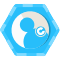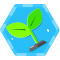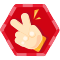如何编写更好的SQL查询：终极指南-第二部分 顶原荐基于集合和程序的方法进行查询

• 查询的程序方法是一种非常类似于编程的方法：你告诉系统需要做些什么以及如何做。例如上一篇文章中的示例，通过执行一个函数然后调用另一个函数来查询数据库，或者使用包含循环、条件和用户定义函数（UDF）的逻辑方式来获得最终查询结果。你会发现通过这种方式，一直在请求一层一层中数据的子集。这种方法也经常被称为逐步或逐行查询。
• 另一种是基于集合的方法，只需指定需要执行的操作。使用这种方法要做的事情就是，指定你想通过查询获得的结果的条件和要求。在检索数据过程中，你不需要关注实现查询的内部机制：数据库引擎会决定最佳的执行查询的算法和逻辑。

查询优化

• 一些软件包功能工具可以生成查询计划的图形表示。
• 其它工具能够为你提供查询计划的文本描述。

EXPLAIN
SELECT *
FROM one_million;
QUERY PLAN
_________________________________________________
Seq Scan on one_million
(cost=0.00..18584.82 rows=1025082 width=36)
(1 row)

ANALYZE one_million;
EXPLAIN
SELECT *
FROM one_million;
QUERY PLAN
_________________________________________________
Seq Scan on one_million
(cost=0.00..18334.00 rows=1000000 width=37)
(1 row)

EXPLAIN ANALYZE
SELECT *
FROM one_million;
QUERY PLAN
___________________________________________________
Seq Scan on one_million
(cost=0.00..18334.00 rows=1000000 width=37)
(actual time=0.015..1207.019 rows=1000000 loops=1)
Total runtime: 2320.146 ms
(2 rows)

EXPLAIN ANALYZE
SELECT *
FROM one_million JOIN half_million
ON (one_million.counter=half_million.counter);
QUERY PLAN
_____________________________________________________________
Hash Join (cost=15417.00..68831.00 rows=500000 width=42)
(actual time=1241.471..5912.553 rows=500000 loops=1)
Hash Cond: (one_million.counter = half_million.counter)
-> Seq Scan on one_million
(cost=0.00..18334.00 rows=1000000 width=37)
(actual time=0.007..1254.027 rows=1000000 loops=1)
-> Hash (cost=7213.00..7213.00 rows=500000 width=5)
(actual time=1241.251..1241.251 rows=500000 loops=1)
Buckets: 4096 Batches: 16 Memory Usage: 770kB
-> Seq Scan on half_million
(cost=0.00..7213.00 rows=500000 width=5)
(actual time=0.008..601.128 rows=500000 loops=1)
Total runtime: 6468.337 ms

CREATE INDEX ON half_million(counter);
EXPLAIN ANALYZE
SELECT *
FROM one_million JOIN half_million
ON (one_million.counter=half_million.counter);
QUERY PLAN
______________________________________________________________
Merge Join (cost=4.12..37650.65 rows=500000 width=42)
(actual time=0.033..3272.940 rows=500000 loops=1)
Merge Cond: (one_million.counter = half_million.counter)
-> Index Scan using one_million_counter_idx on one_million
(cost=0.00..32129.34 rows=1000000 width=37)
(actual time=0.011..694.466 rows=500001 loops=1)
-> Index Scan using half_million_counter_idx on half_million
(cost=0.00..14120.29 rows=500000 width=5)
(actual time=0.010..683.674 rows=500000 loops=1)
Total runtime: 3833.310 ms
(5 rows)葡萄城技术团队powertoolsteam
2017/09/05
0
0

powertoolsteam
2017/09/05
0
0

powertoolsteam
2017/08/29
0
0

powertoolsteam
2017/09/11
0
0

powertoolsteam
2017/09/11
0
0

ERC-777以太坊新代币标准解读

ERC777是一个新的高级代币标准，可以视为ERC20的升级版本，因此它解决了ERC20以及ERC223存在的一些问题，开发者可以根据自己的具体需求进行选型。 1、使用ERC820进行合约注册 有别于ERC20的自...

51分钟前
4
0

code-ortaerc

5
0

[TOC] 1.1.1标签操作v-text&v-html v-text：会把data中绑定的数据值原样输出。 v-html：会把data中值输出，且会自动解析html代码 <!--可以将指定的内容显示到标签体中--><标签 v-text=""></......

8
0
VMware vSphere的两种RDM磁盘

13
0
【AngularJS学习笔记】02 小杂烩及学习总结

16
0